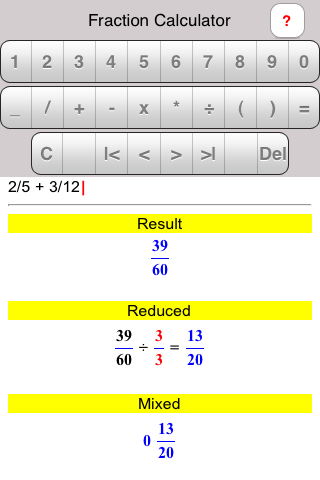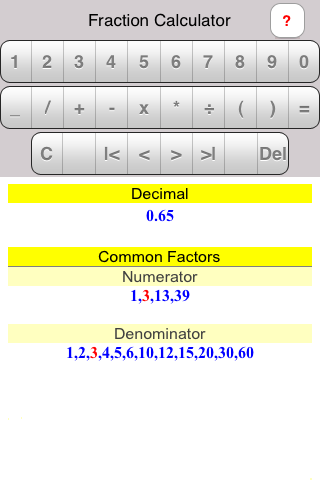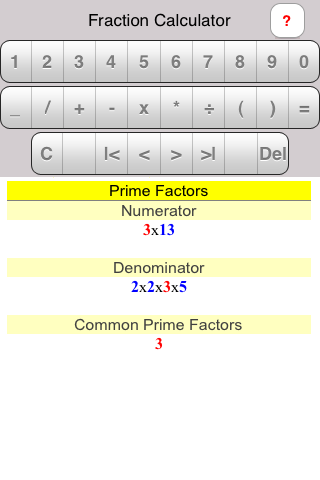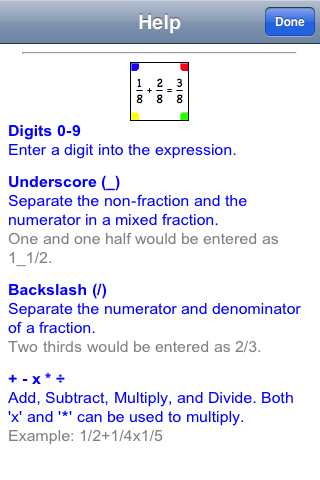Make Math Work
iPhone® and iPod Touch® Fraction Solver
 Home

Welcome to Fraction Solver
Fraction Solver is a no-nonsense easy-to-use application available on the Apple iTunes Store. Use Fraction Solver to perform calculations on fractions. With the free-form data entry you enter your fractional expression as a true fraction, that is, using a numerator-slash-denominator format. It supports algebraic hierarchy and parentheses. The results are displayed in several forms: the raw fraction result,a reduced result, a mixed result, and a decimal equivalent. Additionally the lists of factors and prime factorization are displayed with the common factor highlighted in red.

This is what you would see when calculating 2/5 + 3/12:
••••How To Use Fraction Solver
Using the three rows of buttons in the upper portion of the screen, enter an expression of fractions and finish by touching the "=" button. Your results will be displayed in the lower portion of the screen. These results will extend off the bottom of the screen; flick the display upward to see all of your results.

These are the details of all of the buttons:

The Top Row
These are the digits 0 - 9. Touch any of them to enter digits into your fraction expression.

The Middle Row
These are all mathematical operators for your fraction expression:

_ (Underscore)
Use the underscore to separate the whole number portion of a mixed fraction from the fractional portion. For example, to enter the mixed fraction one and two thirds, enter 1_2/3.

/ (Backslash)
Use the backslash to separate the numerator and the denominator of a fraction. For example, three-fourths would be entered as 3/4.

Use + as the addition operator. To add 1/2 and 3/4, enter 1/2+3/4. If you touch the "=" button you will see a list of results based on the sum of 5/4.

- (Subtraction)
Use - as the subtraction operator. To subtract 1/2 from 3/4, enter 3/4-1/2. If you touch the "=" button you will see a list of results based on the difference of 1/4.

* or x (Multiplication)
Use either * or x as the multiplication operator. To multiply 1/2 and 3/4, enter 1/2*3/4 or 1/2x3/4. If you touch the "=" button you will see a list of results based on the product of 3/8.

÷ (Division)
Use ÷ as the division operator. To divide 3/4 and 1/2, enter 3/4÷1/2. If you touch the "=" button you will see a list of results based on the quotient of 6/4. Remember that the backslash (/) separates a numerator and denominator; it is not a division operator. The expression 3/4 / 1/2 will not yield anything useful.

( ) (Parentheses)
Use ( ) to force the order of operations. Fraction Solver uses standard algebraic hierarchy. You can force the order to suit your entry. Note the difference between 1/4 + 1/3 * 1/2 and (1/4 + 1/3) * 1/2. Without the parentheses, the 1/3 * 1/2 was evaluated first since according to algebraic hierarchy, multiplications and divisions are performed first; the additions and subtractions follow. Using the parentheses forced the 1/4 + 1/3 portion to evaluate first.

= (Equals)
Use = to evaluate your calculation.

The Bottom Row
C
Completely clear the expression.

Space
Add space to the expression. The space does nothing mathematically but it can make the expression easier to read.

|<
Move the cursor to the beginning of the expression.

<
Move the cursor one character to the left.

>
Move the cursor one character to the right.

>|
Move the cursor to the end of the expression.

Del
Clear the character to the left of the cursor (|).

?
Switch to the help screen.

Other Considerations
Fraction Solver does its work using integer math. Because of this, there are some situations that might at first seem like an error occurred, but upon examination, are correct.
1. When doing a division, Fraction Solver will cross-multiply rather than divide. It will use these results without any preliminary reduction in its display of the raw result. For example, if you divide 10/21 by 2/7, you might expect the raw result to be 5/3 since dividing the numerators yields 5 (10 divided by 2) and dividing the denominators yields 3 (21 divided by 7). Instead, the raw result will be 70/42, since 10 multiplied by 7 yields 70 and 21 multiplied by 2 yields 42. 70/42 reduces to 5/3, but it may not have been readily apparent that Fraction Solver cross-multiplied rather than dividing numerators and denominators.
2. When using parentheses to enclose a division in a denominator, Fraction Solver will consider the fractional equivalent and cross-multiply rather than divide. For example, if you entered 1/(6÷3), you might expect an immediate raw result of 1/2. Rather, Fraction Solver will consider this operation as 1/6 x 3/1 to yield a result of 3/6. Again, 3/6 reduced is 1/2, but it may not be readily apparent how Fraction Solver arrived at the preliminary result of 3/6.

Hint
To get information about a fraction such as its reduced form or the common factors without actually performing a calculation, simply enter the fraction and touch the "=" button. For example, to reduce 10/12 enter "1 0 / 1 2 =".

Even More Help
If your question has not been answered you are welcome to email us at support@MakeMathWork.com. Please include:
1. The name of this application: Fraction Solver.
2. A detailed description of the problem.
3. The exact steps to reproduce the problem.
4. A screenshot if possible.